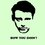# This note has been used to help create the Squeeze Theorem wiki

Squeeze theorem

Assume that functions $$f,\ g,\ h$$ satisfy $g(x)\le f(x)\le h(x)$ and $\lim_{x\to a}g(x)=\lim_{x\to a}h(x)=L.$ Then $\lim_{x\to a}f(x)=L.$

$\boxed{\textbf{Proof}}$

(1) When $L=0$

Assuming that $\forall x\in\textbf{R},\ g(x)=0,\ \lim_{x\to a}h(x)=0$ We have $\forall\varepsilon>0,\ \exists\delta>0:\ 0<|x-a|<\delta\Rightarrow |h(x)|<\varepsilon$ Because $0=g(x)\le f(x)\le h(x)$, so $|f(x)|\le|h(x)|$, which means $\forall\varepsilon>0,\ \exists\delta>0:\ 0<|x-a|<\delta\Rightarrow |f(x)|\le|h(x)|<\varepsilon$ $\Rightarrow\lim_{x\to a}f(x)=0.$

(2) General situation $g(x)\le f(x)\le h(x)\Rightarrow 0\le f(x)-g(x)\le h(x)-g(x)$ When $x\to a$ $h(x)-g(x)=L-L=0$ According to (1), we have $f(x)-g(x)\to 0$ $f(x)=(f(x)-g(x))+g(x)\to 0+L=L.$

$\boxed{Example 1}$ $\lim_{n\to\infty}(\frac{1}{n+1}+\frac{1}{n+\sqrt2}+\cdots+\frac{1}{n+\sqrt n})=?$

$\boxed{Example 2}$ $\lim_{n\to\infty}(\frac{1}{n^2+n+1}+\frac{2}{n^2+n+2}+\cdots +\frac{n}{n^2+n+n})=?$

$\boxed{Solution 1}$ $\text{Let}\ S_n=\frac{1}{n+1}+\frac{1}{n+\sqrt2}+\cdots+\frac{1}{n+\sqrt n},\ \text{then}$ $\frac{n}{n+\sqrt n} $\text{Note that}\ \lim_{n\to\infty}\frac{n}{n+\sqrt n}=\lim_{n\to\infty}\frac{n}{n+1}=1$ $\Rightarrow\lim_{n\to\infty}(\frac{1}{n+1}+\frac{1}{n+\sqrt2}+\cdots+\frac{1}{n+\sqrt n})=1$

$\boxed{Solution 2}$ $\text{Let}\ S_n=\frac{1}{n^2+n+1}+\frac{2}{n^2+n+2}+\cdots +\frac{n}{n^2+n+n},$ $\Rightarrow \frac{1}{n^2+n+n}+\frac{2}{n^2+n+n}+\cdots +\frac{n}{n^2+n+n}$ $<\frac{1}{n^2+n+1}+\frac{2}{n^2+n+2}+\cdots +\frac{n}{n^2+n+n}$ $<\frac{1}{n^2+n+1}+\frac{2}{n^2+n+1}+\cdots +\frac{n}{n^2+n+1}$ $\Rightarrow \frac{\frac{1}{2}n(n+1)}{n^2+n+n} $\text{Note that}\ \lim_{n\to\infty}\frac{\frac{1}{2}n(n+1)}{n^2+n+n}=\lim_{n\to\infty}\frac{\frac{1}{2}n(n+1)}{n^2+n+1}=\frac{1}{2}$ $\Rightarrow\lim_{n\to\infty}(\frac{1}{n^2+n+1}+\frac{2}{n^2+n+2}+\cdots +\frac{n}{n^2+n+n})=\frac{1}{2}$Note by Yin Zhao
7 years, 1 month ago

This discussion board is a place to discuss our Daily Challenges and the math and science related to those challenges. Explanations are more than just a solution — they should explain the steps and thinking strategies that you used to obtain the solution. Comments should further the discussion of math and science.

When posting on Brilliant:

• Use the emojis to react to an explanation, whether you're congratulating a job well done , or just really confused .
• Ask specific questions about the challenge or the steps in somebody's explanation. Well-posed questions can add a lot to the discussion, but posting "I don't understand!" doesn't help anyone.
• Try to contribute something new to the discussion, whether it is an extension, generalization or other idea related to the challenge.

MarkdownAppears as
*italics* or _italics_ italics
**bold** or __bold__ bold
- bulleted- list
• bulleted
• list
1. numbered2. list
1. numbered
2. list
Note: you must add a full line of space before and after lists for them to show up correctly
paragraph 1paragraph 2

paragraph 1

paragraph 2

[example link](https://brilliant.org)example link
> This is a quote
This is a quote
    # I indented these lines
# 4 spaces, and now they show
# up as a code block.

print "hello world"
# I indented these lines
# 4 spaces, and now they show
# up as a code block.

print "hello world"
MathAppears as
Remember to wrap math in $$ ... $$ or $ ... $ to ensure proper formatting.
2 \times 3 $2 \times 3$
2^{34} $2^{34}$
a_{i-1} $a_{i-1}$
\frac{2}{3} $\frac{2}{3}$
\sqrt{2} $\sqrt{2}$
\sum_{i=1}^3 $\sum_{i=1}^3$
\sin \theta $\sin \theta$
\boxed{123} $\boxed{123}$